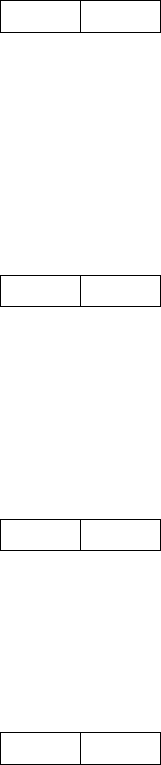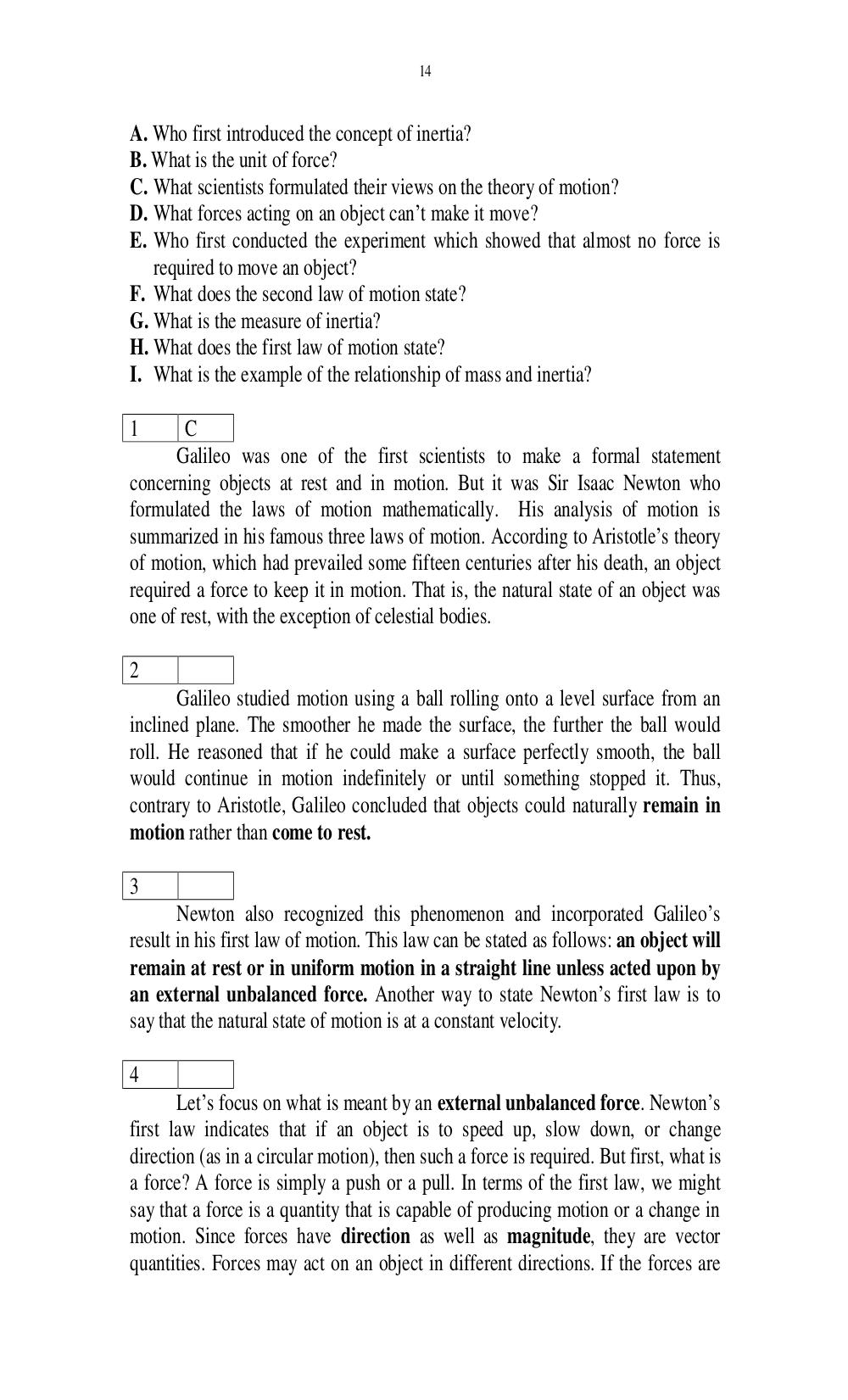# Английский язык. Ильичева Н.А - 14 стр.

Составители:

Рубрика:

• ## Иностранный язык14
A. Who first introduced the concept of inertia?
B. What is the unit of force?
C. What scientists formulated their views on the theory of motion?
D. What forces acting on an object cant make it move?
E. Who first conducted the experiment which showed that almost no force is
required to move an object?
F. What does the second law of motion state?
G. What is the measure of inertia?
H. What does the first law of motion state?
I. What is the example of the relationship of mass and inertia?
1 C
Galileo was one of the first scientists to make a formal statement
concerning objects at rest and in motion. But it was Sir Isaac Newton who
formulated the laws of motion mathematically. His analysis of motion is
summarized in his famous three laws of motion. According to Aristotles theory
of motion, which had prevailed some fifteen centuries after his death, an object
required a force to keep it in motion. That is, the natural state of an object was
one of rest, with the exception of celestial bodies.
2
Galileo studied motion using a ball rolling onto a level surface from an
inclined plane. The smoother he made the surface, the further the ball would
roll. He reasoned that if he could make a surface perfectly smooth, the ball
would continue in motion indefinitely or until something stopped it. Thus,
contrary to Aristotle, Galileo concluded that objects could naturally remain in
motion rather than come to rest.
3
Newton also recognized this phenomenon and incorporated Galileos
result in his first law of motion. This law can be stated as follows: an object will
remain at rest or in uniform motion in a straight line unless acted upon by
an external unbalanced force. Another way to state Newtons first law is to
say that the natural state of motion is at a constant velocity.
4
Lets focus on what is meant by an external unbalanced force. Newtons
first law indicates that if an object is to speed up, slow down, or change
direction (as in a circular motion), then such a force is required. But first, what is
a force? A force is simply a push or a pull. In terms of the first law, we might
say that a force is a quantity that is capable of producing motion or a change in
motion. Since forces have direction as well as magnitude, they are vector
quantities. Forces may act on an object in different directions. If the forces are14

A. Who first introduced the concept of inertia?
B. What is the unit of force?
C. What scientists formulated their views on the theory of motion?
D. What forces acting on an object can’t make it move?
E. Who first conducted the experiment which showed that almost no force is
required to move an object?
F. What does the second law of motion state?
G. What is the measure of inertia?
H. What does the first law of motion state?
I. What is the example of the relationship of mass and inertia?

1       C
Galileo was one of the first scientists to make a formal statement
concerning objects at rest and in motion. But it was Sir Isaac Newton who
formulated the laws of motion mathematically. His analysis of motion is
summarized in his famous three laws of motion. According to Aristotle’s theory
of motion, which had prevailed some fifteen centuries after his death, an object
required a force to keep it in motion. That is, the natural state of an object was
one of rest, with the exception of celestial bodies.

2
Galileo studied motion using a ball rolling onto a level surface from an
inclined plane. The smoother he made the surface, the further the ball would
roll. He reasoned that if he could make a surface perfectly smooth, the ball
would continue in motion indefinitely or until something stopped it. Thus,
contrary to Aristotle, Galileo concluded that objects could naturally remain in
motion rather than come to rest.

3
Newton also recognized this phenomenon and incorporated Galileo’s
result in his first law of motion. This law can be stated as follows: an object will
remain at rest or in uniform motion in a straight line unless acted upon by
an external unbalanced force. Another way to state Newton’s first law is to
say that the natural state of motion is at a constant velocity.

4
Let’s focus on what is meant by an external unbalanced force. Newton’s
first law indicates that if an object is to speed up, slow down, or change
direction (as in a circular motion), then such a force is required. But first, what is
a force? A force is simply a push or a pull. In terms of the first law, we might
say that a force is a quantity that is capable of producing motion or a change in
motion. Since forces have direction as well as magnitude, they are vector
quantities. Forces may act on an object in different directions. If the forces are